Main Content

# Counterparty Credit Risk and CVA

This example shows how to compute the unilateral credit value (valuation) adjustment (CVA) for a bank holding a portfolio of vanilla interest-rate swaps with several counterparties. CVA is the expected loss on an over-the-counter contract or portfolio of contracts due to counterparty default. The CVA for a particular counterparty is defined as the sum over all points in time of the discounted expected exposure at each moment multiplied by the probability that the counterparty defaults at that moment, all multiplied by 1 minus the recovery rate. The CVA formula is:

`$CVA=\left(1-R\right){\int }_{0}^{T}discEE\left(t\right)dPD\left(t\right)$`

Where `R` is the recovery, `discEE` the discounted expected exposure at time t, and `PD` the default probability distribution.

The expected exposure is computed by first simulating many future scenarios of risk factors for the given contract or portfolio. Risk factors can be interest rates, as in this example, but will differ based on the portfolio and can include FX rates, equity or commodity prices, or anything that will affect the market value of the contracts. Once a sufficient set of scenarios has been simulated, the contract or portfolio can be priced on a series of future dates for each scenario. The result is a matrix, or "cube", of contract values.

These prices are converted into exposures after taking into account collateral agreements that the bank might have in place as well as netting agreements, as in this example, where the values of several contracts may offset each other, lowering their total exposure.

The contract values for each scenario are discounted to compute the discounted exposures. The discounted expected exposures can then be computed by a simple average of the discounted exposures at each simulation date.

Finally, counterparty default probabilities are typically derived from credit default swap (CDS) market quotes and the CVA for the counterparty can be computed according to the above formula. Assume that a counterparty default is independent of its exposure (no wrong-way risk).

This example demonstrates a portfolio of vanilla interest-rate swaps with the goal of computing the CVA for a particular counterparty.

### Read Swap Portfolio

The portfolio of swaps is close to zero value at time t = `0`. Each swap is associated with a counterparty and may or may not be included in a netting agreement.

```% Read swaps from spreadsheet swapFile = 'cva-swap-portfolio.xls'; swaps = readtable(swapFile,'Sheet','Swap Portfolio'); swaps.LegType = [swaps.LegType ~swaps.LegType]; swaps.LegRate = [swaps.LegRateReceiving swaps.LegRatePaying]; swaps.LegReset = ones(size(swaps,1),1); numSwaps = size(swaps,1);```

For more information on the swap parameters for `CounterpartyID` and `NettingID`, see `creditexposures`. For more information on the swap parameters for `Principal`, `Maturity`, `LegType`, `LegRate`, `LatestFloatingRate`, `Period`, and `LegReset`, see `swapbyzero`.

### Create RateSpec from the Interest-Rate Curve

```Settle = datenum('14-Dec-2007'); Tenor = [3 6 12 5*12 7*12 10*12 20*12 30*12]'; ZeroRates = [0.033 0.034 0.035 0.040 0.042 0.044 0.048 0.0475]'; ZeroDates = datemnth(Settle,Tenor); Compounding = 2; Basis = 0; RateSpec = intenvset('StartDates', Settle,'EndDates', ZeroDates, ... 'Rates', ZeroRates,'Compounding',Compounding,'Basis',Basis); figure; plot(ZeroDates, ZeroRates, 'o-'); xlabel('Date'); datetick('keeplimits'); ylabel('Zero rate'); grid on; title('Yield Curve at Settle Date');```### Set Changeable Simulation Parameters

You can vary the number of simulated interest-rate scenarios that you generate. This example sets the simulation dates to be more frequent at first, then turning less frequent further in the future.

```% Number of Monte Carlo simulations numScenarios = 1000; % Compute monthly simulation dates, then quarterly dates later. simulationDates = datemnth(Settle,0:12); simulationDates = [simulationDates datemnth(simulationDates(end),3:3:74)]'; numDates = numel(simulationDates);```

### Compute Floating Reset Dates

For each simulation date, compute previous floating reset date for each swap.

```floatDates = cfdates(Settle-360,swaps.Maturity,swaps.Period); swaps.FloatingResetDates = zeros(numSwaps,numDates); for i = numDates:-1:1 thisDate = simulationDates(i); floatDates(floatDates > thisDate) = 0; swaps.FloatingResetDates(:,i) = max(floatDates,[],2); end```

### Setup Hull-White Single Factor Model

The risk factor that is simulated to value the contracts is the zero curve. For this example, you model the interest-rate term structure using the one-factor Hull-White model. This is a model of the short rate and is defined as:

`$dr=\left[\theta \left(t\right)-ar\right]dt+\sigma dz$`

where

• $dr$: Change in the short rate after a small change in time, $dt$

• $a$: Mean reversion rate

• $\sigma$: Volatility of the short rate

• $dz$: A Weiner process (a standard normal process)

• $\theta \left(t\right)$: Drift function defined as:

`$\theta \left(t\right)={F}_{t}\left(0,t\right)+aF\left(0,t\right)+\frac{{\sigma }^{2}}{2a}\left(1-{e}^{-2at}\right)$`

$F\left(0,t\right)$: Instantaneous forward rate at time $t$

${F}_{t}\left(0,t\right)$: Partial derivative of $F$ with respect to time

Once you have simulated a path of the short rate, generate a full yield curve at each simulation date using the formula:

`$R\left(t,T\right)=-\frac{1}{\left(T-t\right)}\mathrm{ln}A\left(t,T\right)+\frac{1}{\left(T-t\right)}B\left(t,T\right)r\left(t\right)$`

`$\mathrm{ln}A\left(t,T\right)=\mathrm{ln}\frac{P\left(0,T\right)}{P\left(0,t\right)}+B\left(t,T\right)F\left(0,t\right)-\frac{1}{4{a}^{3}}{\sigma }^{2}\left({e}^{-aT}-{e}^{-at}{\right)}^{2}\left({e}^{2at}-1\right)$`

`$B\left(t,T\right)=\frac{1-{e}^{-a\left(T-t\right)}}{a}$`

$R\left(t,T\right)$: Zero rate at time $t$ for a period of $T-t$

$P\left(t,T\right)$: Price of a zero coupon bond at time $t$ that pays one dollar at time $T$

Each scenario contains the full term structure moving forward through time, modeled at each of our selected simulation dates.

Refer to the Calibrating Hull-White Model Using Market Data example in the Financial Instruments Toolbox™ Users' Guide for more details on Hull-White one-factor model calibration.

```Alpha = 0.2; Sigma = 0.015; hw1 = HullWhite1F(RateSpec,Alpha,Sigma);```

### Simulate Scenarios

For each scenario, simulate the future interest-rate curve at each valuation date using the Hull-White one-factor interest-rate model.

```% Use reproducible random number generator (vary the seed to produce % different random scenarios). prevRNG = rng(0, 'twister'); dt = diff(yearfrac(Settle,simulationDates,1)); nPeriods = numel(dt); scenarios = hw1.simTermStructs(nPeriods, ... 'nTrials',numScenarios, ... 'deltaTime',dt); % Restore random number generator state rng(prevRNG); % Compute the discount factors through each realized interest rate % scenario. dfactors = ones(numDates,numScenarios); for i = 2:numDates tenorDates = datemnth(simulationDates(i-1),Tenor); rateAtNextSimDate = interp1(tenorDates,squeeze(scenarios(i-1,:,:)), ... simulationDates(i),'linear','extrap'); % Compute D(t1,t2) dfactors(i,:) = zero2disc(rateAtNextSimDate, ... repmat(simulationDates(i),1,numScenarios),simulationDates(i-1),-1,3); end dfactors = cumprod(dfactors,1);```

### Inspect a Scenario

Create a surface plot of the yield curve evolution for a particular scenario.

```i = 20; figure; surf(Tenor, simulationDates, scenarios(:,:,i)) axis tight datetick('y','mmmyy'); xlabel('Tenor (Months)'); ylabel('Observation Date'); zlabel('Rates'); ax = gca; ax.View = [-49 32]; title(sprintf('Scenario %d Yield Curve Evolution\n',i));```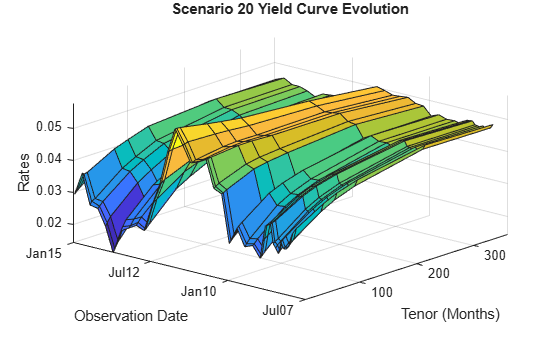### Compute Mark to Market Swap Prices

For each scenario the swap portfolio is priced at each future simulation date. Prices are computed using a price approximation function, `hswapapprox`. It is common in CVA applications to use simplified approximation functions when pricing contracts due to the performance requirements of these Monte Carlo simulations.

Since the simulation dates do not correspond to the swaps cash flow dates (where the floating rates are reset) estimate the latest floating rate with the 1-year rate (all swaps have period 1 year) interpolated between the nearest simulated rate curves.

The swap prices are then aggregated into a "cube" of values which contains all future contract values at each simulation date for each scenario. The resulting cube of contract prices is a 3-dimensional matrix where each row represents a simulation date, each column a contract, and each "page" a different simulated scenario.

```% Compute all mark-to-market values for this scenario. Use an % approximation function here to improve performance. values = hcomputeMTMValues(swaps,simulationDates,scenarios,Tenor);```

### Inspect Scenario Prices

Create a plot of the evolution of all swap prices for a particular scenario.

```i = 32; figure; plot(simulationDates, values(:,:,i)); datetick; ylabel('Mark-To-Market Price'); title(sprintf('Swap prices along scenario %d', i));```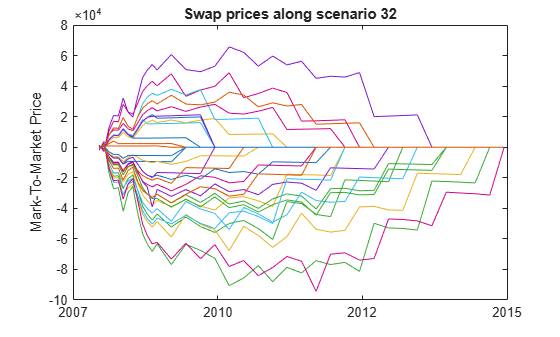### Visualize Simulated Portfolio Values

Plot the total portfolio value for each scenario of the simulation. As each scenario moves forward in time, the values of the contracts move up or down depending on how the modeled interest-rate term structure changes. As the swaps get closer to maturity, their values will begin to approach zero since the aggregate value of all remaining cash flows will decrease after each cash flow date.

```% View portfolio value over time figure; totalPortValues = squeeze(sum(values, 2)); plot(simulationDates,totalPortValues); title('Total MTM Portfolio Value for All Scenarios'); datetick('x','mmmyy') ylabel('Portfolio Value (\$)') xlabel('Simulation Dates')```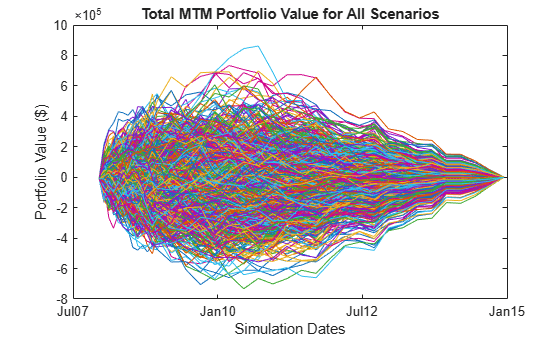### Compute Exposure by Counterparty

The exposure of a particular contract (i) at time t is the maximum of the contract value (Vi) and `0`:

`${E}_{i}\left(t\right)=\mathrm{max}\left\{{V}_{i}\left(t\right),0\right\}$`

And the exposure for a particular counterparty is simply a sum of the individual contract exposures:

`${E}_{cp}\left(t\right)=\sum {E}_{i}\left(t\right)=\sum \mathrm{max}\left\{{V}_{i}\left(t\right),0\right\}$`

In the presence of netting agreements, however, contracts are aggregated together and can offset each other. Therefore the total exposure of all contracts in a netting agreement is:

`${E}_{na}\left(t\right)=\mathrm{max}\left\{\sum {V}_{i}\left(t\right),0\right\}$`

Compute these exposures for the entire portfolio as well as each counterparty at each simulation date using the `creditexposures` function.

Unnetted contracts are indicated using a `NaN` in the `NettingID` vector. Exposure of an unnetted contract is equal to the market value of the contract if it has positive value, otherwise it is zero.

Contracts included in a netting agreement have their values aggregated together and can offset each other. See the references for more details on computing exposure from mark-to-market contract values.

```[exposures, expcpty] = creditexposures(values,swaps.CounterpartyID, ... 'NettingID',swaps.NettingID);```

Plot the total portfolio exposure for each scenario in our simulation. Similar to the plot of contract values, the exposures for each scenario will approach zero as the swaps mature.

```% View portfolio exposure over time figure; totalPortExposure = squeeze(sum(exposures,2)); plot(simulationDates,totalPortExposure); title('Portfolio Exposure for All Scenarios'); datetick('x','mmmyy') ylabel('Exposure (\$)') xlabel('Simulation Dates')```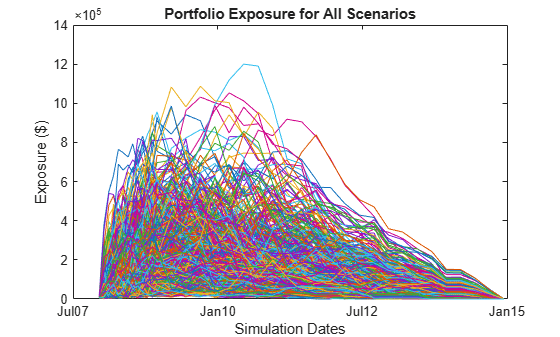### Exposure Profiles

Several exposure profiles are useful when analyzing the potential future exposure of a bank to a counterparty. Here you can compute several (non-discounted) exposure profiles per counterparty, as well as, for the entire portfolio.

• `PFE` (Potential Future Exposure): A high percentile (95%) of the distribution of exposures at any particular future date (also called Peak Exposure (PE))

• `MPFE` (Maximum Potential Future Exposure): The maximum PFE across all dates

• `EE` : (Expected Exposure): The mean (average) of the distribution of exposures at each date

• `EPE` (Expected Positive Exposure): Weighted average over time of the expected exposure

• `EffEE `(Effective Expected Exposure): The maximum expected exposure at any time, t, or previous time

• `EffEPE` (Effective Expected Positive Exposure): The weighted average of the effective expected exposure

For further definitions, see for example the Basel II document in references.

```% Compute entire portfolio exposure portExposures = sum(exposures,2); % Compute exposure profiles for each counterparty and entire portfolio cpProfiles = exposureprofiles(simulationDates,exposures); portProfiles = exposureprofiles(simulationDates,portExposures);```

Visualize the exposure profiles, first for the entire portfolio, then for a particular counterparty.

```% Visualize portfolio exposure profiles figure; plot(simulationDates,portProfiles.PFE, ... simulationDates,portProfiles.MPFE * ones(numDates,1), ... simulationDates,portProfiles.EE, ... simulationDates,portProfiles.EPE * ones(numDates,1), ... simulationDates,portProfiles.EffEE, ... simulationDates,portProfiles.EffEPE * ones(numDates,1)); legend({'PFE (95%)','Max PFE','Exp Exposure (EE)','Time-Avg EE (EPE)', ... 'Max past EE (EffEE)','Time-Avg EffEE (EffEPE)'}) datetick('x','mmmyy') title('Portfolio Exposure Profiles'); ylabel('Exposure (\$)') xlabel('Simulation Dates')```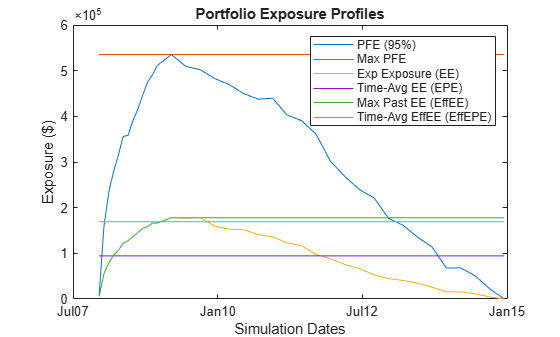Visualize exposure profiles for a particular counterparty.

```cpIdx = find(expcpty == 5); figure; plot(simulationDates,cpProfiles(cpIdx).PFE, ... simulationDates,cpProfiles(cpIdx).MPFE * ones(numDates,1), ... simulationDates,cpProfiles(cpIdx).EE, ... simulationDates,cpProfiles(cpIdx).EPE * ones(numDates,1), ... simulationDates,cpProfiles(cpIdx).EffEE, ... simulationDates,cpProfiles(cpIdx).EffEPE * ones(numDates,1)); legend({'PFE (95%)','Max PFE','Exp Exposure (EE)','Time-Avg EE (EPE)', ... 'Max past EE (EffEE)','Time-Avg EffEE (EffEPE)'}) datetick('x','mmmyy','keeplimits') title(sprintf('Counterparty %d Exposure Profiles',cpIdx)); ylabel('Exposure (\$)') xlabel('Simulation Dates')```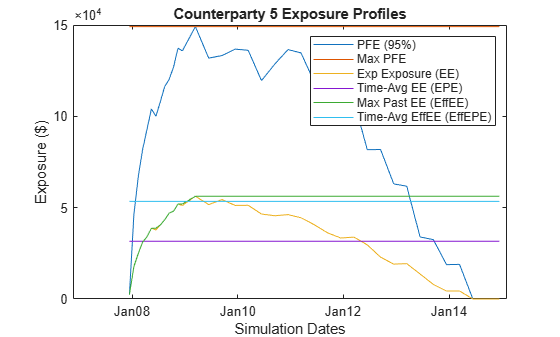### Discounted Exposures

Compute the discounted expected exposures using the discount factors from each simulated interest-rate scenario. The discount factor for a given valuation date in a given scenario is the product of the incremental discount factors from one simulation date to the next, along with the interest-rate path of that scenario.

```% Get discounted exposures per counterparty, for each scenario discExp = zeros(size(exposures)); for i = 1:numScenarios discExp(:,:,i) = bsxfun(@times,dfactors(:,i),exposures(:,:,i)); end % Discounted expected exposure discProfiles = exposureprofiles(simulationDates,discExp, ... 'ProfileSpec','EE');```

Plot the discounted expected exposures for the aggregate portfolio as well as for each counterparty.

```% Aggregate the discounted EE for each counterparty into a matrix discEE = [discProfiles.EE]; % Portfolio discounted EE figure; plot(simulationDates,sum(discEE,2)) datetick('x','mmmyy','keeplimits') title('Discounted Expected Exposure for Portfolio'); ylabel('Discounted Exposure (\$)') xlabel('Simulation Dates')```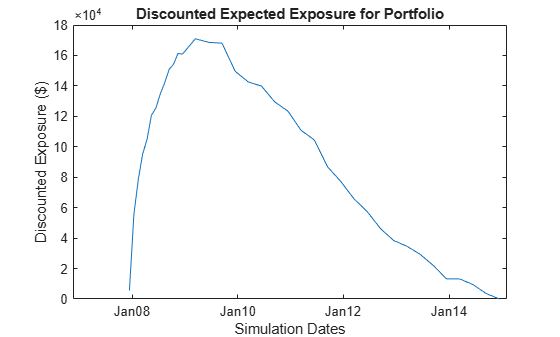```% Counterparty discounted EE figure; plot(simulationDates,discEE) datetick('x','mmmyy','keeplimits') title('Discounted Expected Exposure for Each Counterparty'); ylabel('Discounted Exposure (\$)') xlabel('Simulation Dates')```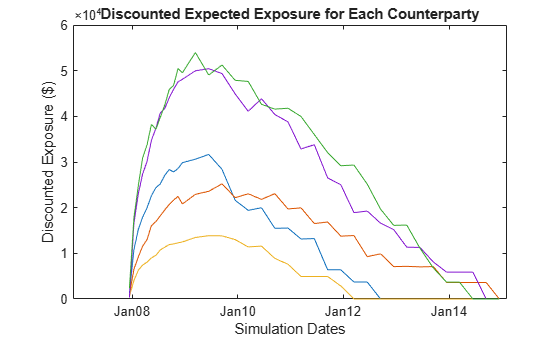### Calibrating Probability of Default Curve for Each Counterparty

The default probability for a given counterparty is implied by the current market spreads of the counterparty's CDS. Use the function `cdsbootstrap` to generate the cumulative probability of default at each simulation date.

```% Import CDS market information for each counterparty CDS = readtable(swapFile,'Sheet','CDS Spreads'); disp(CDS);```
``` Date cp1 cp2 cp3 cp4 cp5 _____________ ___ ___ ___ ___ ___ {'3/20/2008'} 140 85 115 170 140 {'3/20/2009'} 185 120 150 205 175 {'3/20/2010'} 215 170 195 245 210 {'3/20/2011'} 275 215 240 285 265 {'3/20/2012'} 340 255 290 320 310 ```
```CDSDates = datenum(CDS.Date); CDSSpreads = table2array(CDS(:,2:end)); ZeroData = [RateSpec.EndDates RateSpec.Rates]; % Calibrate default probabilities for each counterparty DefProb = zeros(length(simulationDates), size(CDSSpreads,2)); for i = 1:size(DefProb,2) probData = cdsbootstrap(ZeroData, [CDSDates CDSSpreads(:,i)], ... Settle, 'probDates', simulationDates); DefProb(:,i) = probData(:,2); end % Plot of the cumulative probability of default for each counterparty. figure; plot(simulationDates,DefProb) title('Default Probability Curve for Each Counterparty'); xlabel('Date'); grid on; ylabel('Cumulative Probability') datetick('x','mmmyy') ylabel('Probability of Default') xlabel('Simulation Dates')```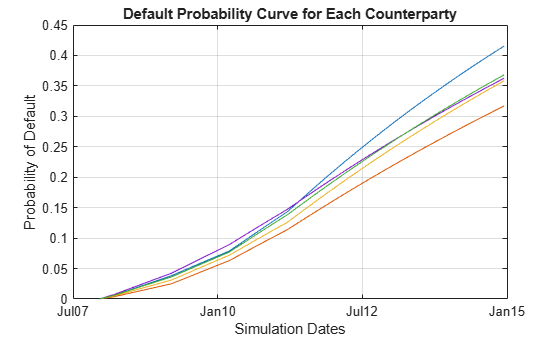### CVA Computation

The Credit Value (Valuation) Adjustment (CVA) formula is:

`$CVA=\left(1-R\right){\int }_{0}^{T}discEE\left(t\right)dPD\left(t\right)$`

Where `R` is the recovery, `discEE` the discounted expected exposure at time t, and `PD` the default probability distribution. This assumes the exposure is independent of default (no wrong-way risk), and it also assumes that the exposures were obtained using risk-neutral probabilities.

Approximate the integral with a finite sum over the valuation dates as:

`$CVA\left(approx\right)=\left(1-R\right)\sum _{i=2}^{n}discEE\left({t}_{i}\right)\left(PD\left({t}_{i}\right)-PD\left({t}_{i-1}\right)\right)$`

where `t_1` is today's date, `t_2`, ...,`t`_n the future valuation dates.

Assume that the CDS information corresponds to the counterparty with index `cpIdx`. The computed CVA is the present market value of our credit exposure to counterparty `cpIdx`. For this example, set the recovery rate at 40%.

```Recovery = 0.4; CVA = (1-Recovery) * sum(discEE(2:end,:) .* diff(DefProb)); for i = 1:numel(CVA) fprintf('CVA for counterparty %d = \$%.2f\n',i,CVA(i)); end```
```CVA for counterparty 1 = \$2229.38 CVA for counterparty 2 = \$2498.71 CVA for counterparty 3 = \$918.96 CVA for counterparty 4 = \$5521.83 CVA for counterparty 5 = \$5883.77 ```
```figure; bar(CVA); title('CVA for each counterparty'); xlabel('Counterparty'); ylabel('CVA \$'); grid on;```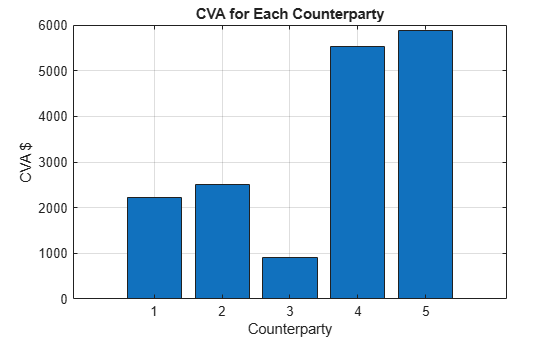### References

1. Pykhtin, Michael, and Steven Zhu, A Guide to Modeling Counterparty Credit Risk, GARP, July/August 2007, issue 37, pp. 16-22.

2. Pykhtin, Michael, and Dan Rosen, Pricing Counterparty Risk at the Trade Level and CVA, 2010.

3. Basel II: https://www.bis.org/publ/bcbs128.pdf page 256.

Download ebook